Question

# A gaseous mixture contains 450.0 Torr H2(g), 372.5 Torr N2(g), and 88.1 Torr Ar(g). Calculate the...

A gaseous mixture contains 450.0 Torr H2(g), 372.5 Torr N2(g), and 88.1 Torr Ar(g). Calculate the mole fraction, χ, of each of these gases.

The mole fraction of each of the given gases in the gaseous mixture can be calculated asXi = P​​​​​​i / P​​​​total ...........(1)

​​​​P​​i = pressure of individual gases (Given)

P​​​​​total = total pressure of the gaseous mixture

Therefore

P​​​​​​total= P​​​​​​N2 + P​​​​​​H2 + P​​​​​​Ar

= (372.5 torr) + ( 450.0 torr) + (88.1 torr)

= 910.6 torr

Therefore, using equation (1) we get,

X​​​​​​H2 = 450.0/ 910.6

= 0.49

X​​​​​​N2​​​​​​​​ = 372.5/ 910.6

= 0.41

X​​​​​​Ar = 88.1/ 910.6

= 0.09

#### Earn Coins

Coins can be redeemed for fabulous gifts.

Similar Homework Help Questions
• ### A gaseous mixture contains 410.0 Torr H2(g), 337.9 Torr N2(g), and 80.9 Torr Ar(g). Calculate the...

A gaseous mixture contains 410.0 Torr H2(g), 337.9 Torr N2(g), and 80.9 Torr Ar(g). Calculate the mole fraction, ?, of each of these gases. H2, N2.Ar

• ### A gaseous mixture contains 415.0 tour H2 (g), Torr N2 (g), and 92.1 Torr Ar (g)....

A gaseous mixture contains 415.0 tour H2 (g), Torr N2 (g), and 92.1 Torr Ar (g). Caluclate the mole fraction, x, of each of these gases. XH2=?, XN2=?, XAr=?

• ### A gaseous mixture contains 438.0 Torr H2(g), 379.9 Torr N, (g), and 71.5 Torr Ar(g). Calculate...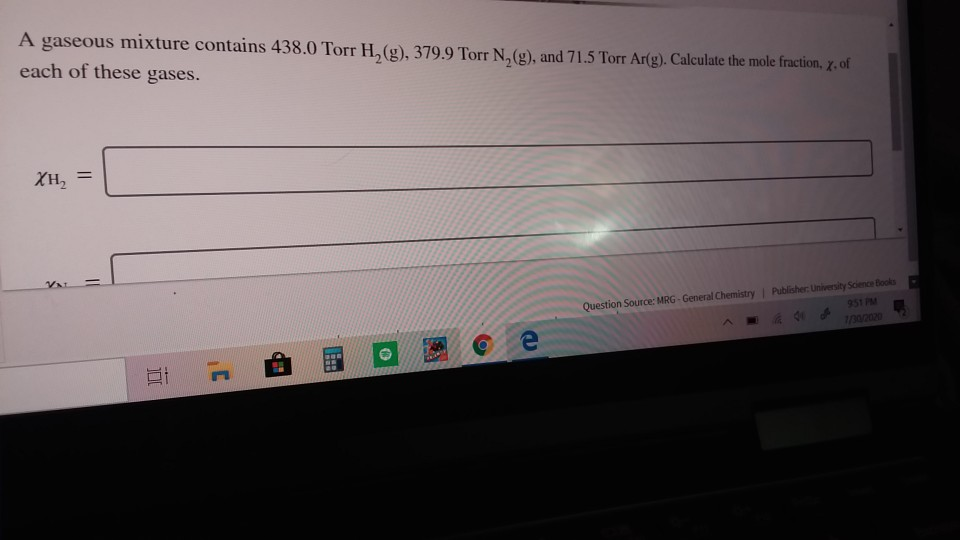A gaseous mixture contains 438.0 Torr H2(g), 379.9 Torr N, (g), and 71.5 Torr Ar(g). Calculate the mole fraction, X, of each of these gases. ΧΗ, Question Source: MRG - General Chemistry | Publisher: University Science Books 951 PM 1/30/2020 се HE UL XH, = XN₂ = = XAr Question Source: MRG - General Chemistry Publishermen som e BE C

• ### A gaseous mixture contains 406.0 Torr H.(g), 341.7 Torr N, (g), and 89.5 Torr Ar(g). Calculate...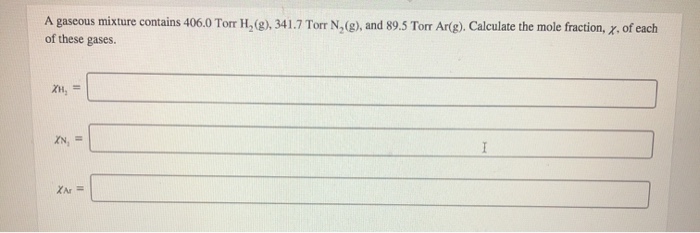A gaseous mixture contains 406.0 Torr H.(g), 341.7 Torr N, (g), and 89.5 Torr Ar(g). Calculate the mole fraction, x, of each of these gases. XH, = IN

• ### A gaseous mixture contains 426.0 Torr H,(g), 384.1 Torr N,(g), and 84.5 Torr Ar(g). Calculate the...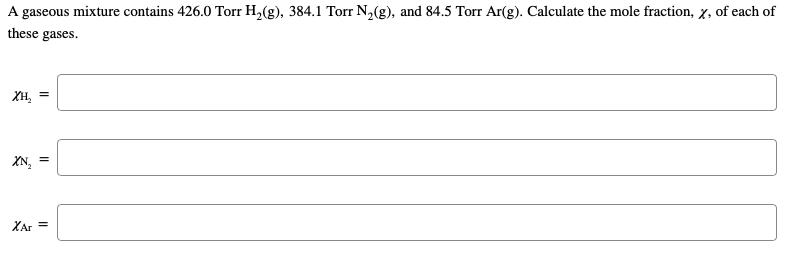A gaseous mixture contains 426.0 Torr H,(g), 384.1 Torr N,(g), and 84.5 Torr Ar(g). Calculate the mole fraction, ^, of each of these gases Хн, XN XAr

• ### A gaseous mixture contains 406.0 Torr of H_2(g), 347.9 Torr of N_2 (g), and 83.1 Torr...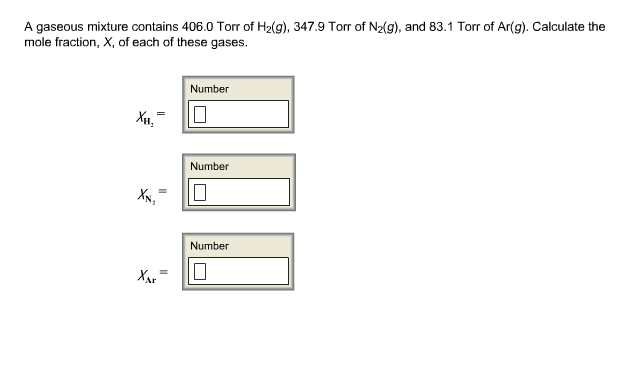A gaseous mixture contains 406.0 Torr of H_2(g), 347.9 Torr of N_2 (g), and 83.1 Torr of Ar(g). Calculate the mole fraction, X, of each of these gases. X_H_2 = X_N_2 = X_Ar =

• ### A gaseous mixture contains 0.540 bar H, (g), 0.457 bar N,(g), and 0.087 bar Ar(g). Calculate...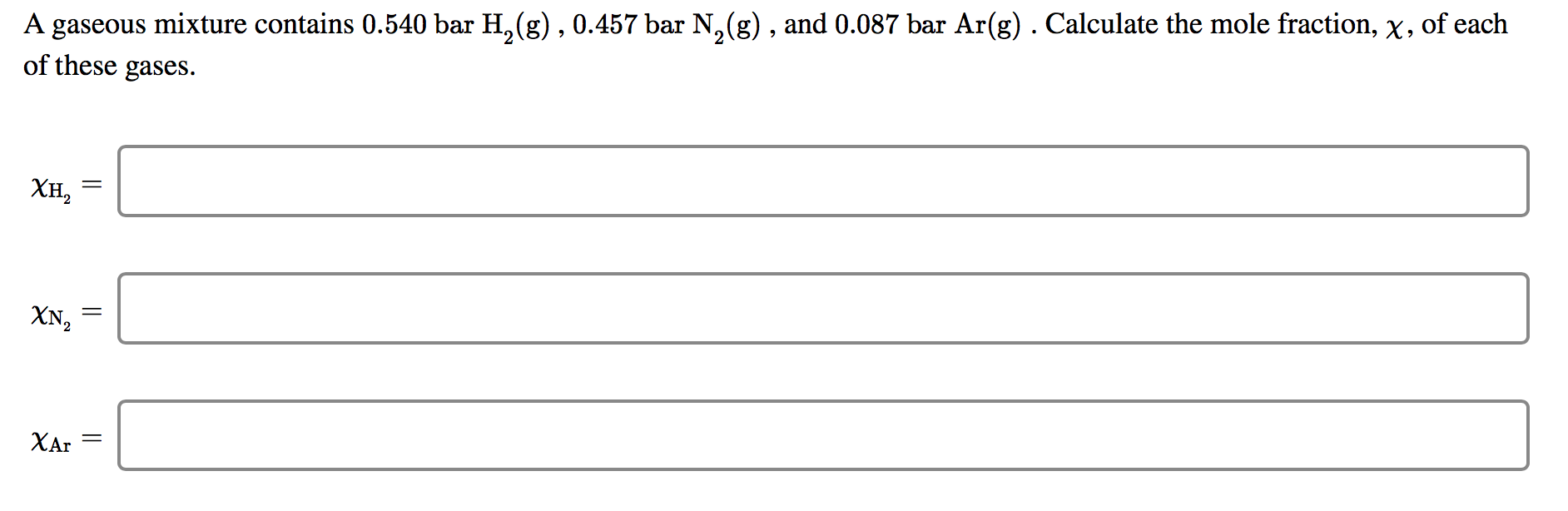A gaseous mixture contains 0.540 bar H, (g), 0.457 bar N,(g), and 0.087 bar Ar(g). Calculate the mole fraction, X, of each of these gases. XH, = XN, = A gaseous mixture of N, and O, has a total pressure of 2.660 bar . If the partial pressure of N, is 1.540 bar , what is the partial pressure of 0,? Pon = bar

• ### A gas mixture contains 9.60 g of N2, 2.80 g of H2 and 13.8 g of...

A gas mixture contains 9.60 g of N2, 2.80 g of H2 and 13.8 g of CH4. What is thr mole fraction of H2 in the mixture? A gas mixture contains 14.0 g of N2, 2.40 g of H2 and 19.4 g of CH4. What is the mole fraction of CH4 in the mixture?

• ### < Question 4 of 23 > A gaseous mixture contains 0.570 bar H, (g), 0.485 bar...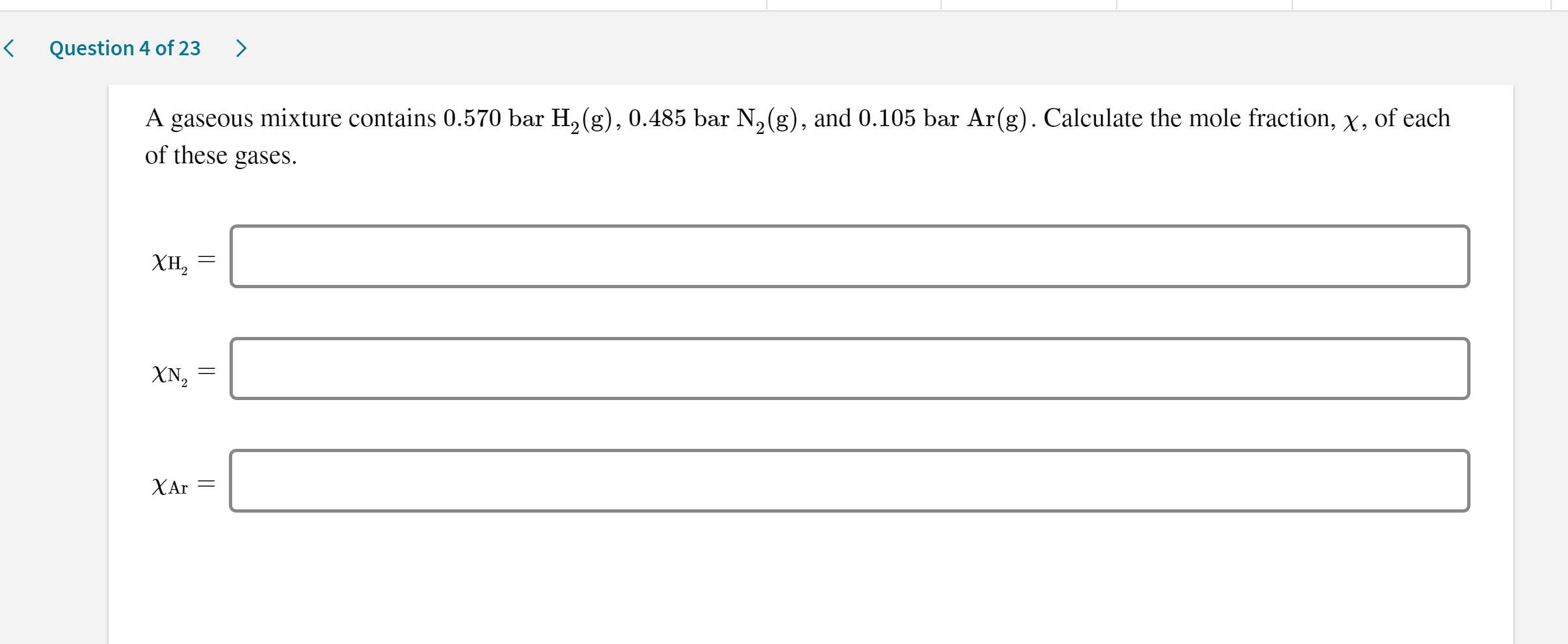< Question 4 of 23 > A gaseous mixture contains 0.570 bar H, (g), 0.485 bar N, (g), and 0.105 bar Ar(g). Calculate the mole fraction, X, of each of these gases. XH, = XA = |

• ### 1st attempt A gas mixture contains 14.0 g of N2, 2.40 g of H2, and 19.4...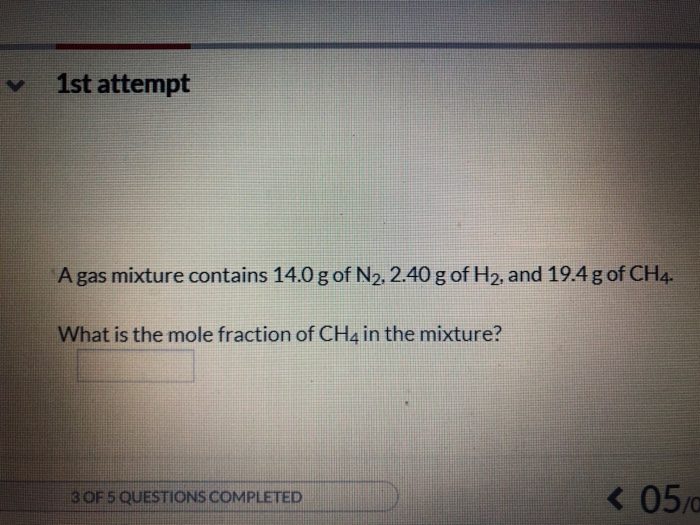1st attempt A gas mixture contains 14.0 g of N2, 2.40 g of H2, and 19.4 g of CH4 What is the mole fraction of CH4 in the mixture? < 05/0 3 OF 5 QUESTIONS COMPLETED A gas mixture contains 9.60 g of N2, 2.80 gof H2, and 13.8 g of CH4. What is the mole fraction of H2 in the mixture?